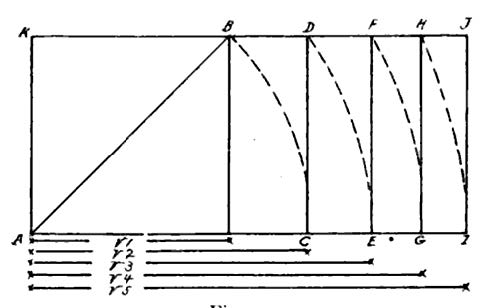# Gauges of a pre-Genesis Lost World are UncoveredGauges of a Lost World are found in an  Isosceles Right Triangle . The shorter legs in this triangle are each 12 British inches in length. A foot long ruler graphically illustrates the length.  Why did I say also? At the turn of the 1900’s Jay Hambidge made a significant discovery. He discovered an ancient canon of measures.  They were derived from a square that measured one Egyptian remen on each side (1.2165 feet). The quote of measures below is  from, Peace Can Be Uncovered by Discovering the Distant Past.  This is the title of my post on DSOworks.com. Currently I have over 240 posts.

Jay Hambidge multiplied the  remen by the square roots of 2,3,4,5,etc…… He found that:

• 1.2165 x square root of 2 =1.72 feet.One royal cubit
• 1.2165 x square root of   3 =2.107 feet. One Palestinian cubit.
• 1.2165 x square root of 4 = 2.433 feet. One Roman pace.
• 1.2165 x square root of 5 = 2.72 feet, One megalithic yard.
• 1.2165 x square root of 6 =  2.98 feet (virtually one British yard).

These same measure labels are close to duplication the isosceles right triangle in the featured picture.Even the gauge of a  classical caliper can also duplicate a multitude of measurements derived from the British 12 inch foot in the remote past.The half diagonal of the initial square, AKB and 4th point,( the right end of line V1,)  is 1.118 tones longer than any side. Each side of the initial square gauges a remen of 1,2165 feet.  The half diagonal is not in this particular  illustration. The half diagonal is elaborated on in City of Revelation by John Michell. Above picture is the  Jay Hambidge drawing of the dynamic rectangle from “The Elements of Dynamic Symmetry,” Dover Art Instruction, 1926. Lines  BC and AC in the Golden Triangle are both 12 British inches. The hypotenuse becomes longer by the   √2 = 16.97 inches.  Next, draw the altitude of this triangle. This is from corner “C” to the line that bisects AB in the featured picture. We’ll call this point “D”. It is virtually 8.5 inches or ½ the length of the hypotenuse. .   We have the following figures for comparison to the Hambidge model:

• AB + BD = 1.707 feet. Hambidge’s figure is 1.718 feet which is hishorter Egyptian cubit
• AB + AC = 2.707 feet. Hambidge’s is 2.72 feet which is his  megalithic yard.
• CD + CA = 1.207 feet. Hambidge’s is  1.2165 feet which is the Egyptian remen.
• Most remarkable: The perimeter around the triangle is gauges 3.41 feet. This is the Central American measure of the Hunab. I have blogged about how this measure most likely preceded the formal creation in Genesis. It is not in Jay Hambidge’s canon. It equates by gematria (341).  Aleph, the 1st letter= 1. Mem, the second letter = 40. Shin, the third letter = 300. Thus 1 + 40 + 300 = 341. Rabbi Aryeh Kaplan disucsses Emesh in Sefer Yitzirah: The Book of Creation-in Theory and Practice. Chapter 3, section 2.
• Strong’s Concordance
emesh: yesterday

Original Word: אָ֫מֶשׁ